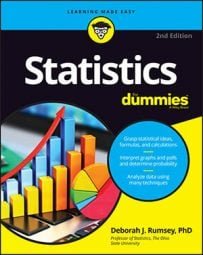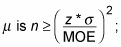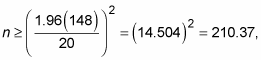##### Statistics For DummiesThe margin of error of a confidence interval (CI) is affected by the size of the statistical sample; as the size increases, the margin of error decreases. Looking at this the other way around, if you want a smaller margin of error (and doesn’t everyone?), you need a larger sample size.

Suppose you are getting ready to do your own survey to estimate a population mean; wouldn’t it be nice to see ahead of time what sample size you need to get the margin of error you want? Thinking ahead will save you money and time and it will give you results you can live with in terms of the margin of error — you won’t have any surprises later.

The formula for the sample size required to get a desired margin of error (MOE) when you are doing a confidence interval foralways round up the sample size no matter what decimal value you get. (For example, if your calculations give you 126.2 people, you can’t just have 0.2 of a person — you need the whole person, so include him by rounding up to 127.)

In this formula, MOE is the number representing the margin of error you want, and z* is the z*-value corresponding to your desired confidence level (from the below table; most people use 1.96 for a 95% confidence interval).

Confidence Level z*-value z*-values for Various Confidence Levels 80% 1.28 90% 1.645 (by convention) 95% 1.96 98% 2.33 99% 2.58
Note that these values are taken from the standard normal (Z-) distribution. The area between each z* value and the negative of that z* value is the confidence percentage (approximately). For example, the area between z*=1.28 and z=-1.28 is approximately 0.80. Hence this chart can be expanded to other confidence percentages as well. The chart shows only the confidence percentages most commonly used.

If the population standard deviation (σ) is unknown, you can put in a worst-case scenario guess for it or run a pilot study (a small trial study) ahead of time, find the standard deviation of the sample data (s), and use that number. This can be risky if the sample size is very small because it’s less likely to reflect the whole population; try to get the largest trial study that you can, and/or make a conservative estimate for (σ).

Often a small trial study is worth the time and effort. Not only will you get an estimate for (σ) to help you determine a good sample size, but you may also learn about possible problems in your data collection.

## Estimating a population mean example

Here’s an example where you need to calculate n to estimate a population mean. Suppose you want to estimate the average number of songs college students store on their portable devices. You want the margin of error to be no more than plus or minus 20 songs. You want a 95 percent confidence interval. How many students should you sample?

Because you want a 95 percent CI, z* is 1.96 (found in the above table); you know your desired MOE is 20. Now you need a number for the population standard deviation (σ). This number is not known, so you do a pilot study of 35 students and find the standard deviation (s) for the sample is 148 songs — use this number as a substitute for (σ).

Using the sample size formula, you calculate the sample size you need iswhich you round up to 211 students (you always round up when calculating n). So you need to take a random sample of at least 211 college students in order to have a margin of error in the number of stored songs of no more than 20. That’s why you see a greater-than-or-equal-to sign in the formula here.

You always round up to the nearest integer when calculating sample size, no matter what the decimal value of your result is (for example, 0.37). That’s because you want the margin of error to be no more than what you stated. If you round down when the decimal value is under .50 (as you normally do in other math calculations), your MOE will be a little larger than you wanted.